# Linear inequalities

## Summary and examples

Solving an inequality is largely the same as solving a linear equation (for more details and assignments see Linear equations)

An example of a linear inequality is: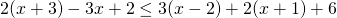The question is to determine for which values ofthis inequality holds.
To solve this inequality, we proceed as follows.

1. First remove the parentheses: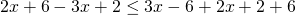2. Next, in both the left- and right-hand side take the's and the constants together: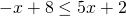3. Now we take's to the left-hand side and the constants to the right-hand side: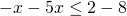resulting in: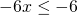So far, the procedure is more or less identical to the solution of linear equations. However, there is also an important difference. When this inequality would have been an equation, we could divide both sides by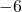. We can do the same with inequalities but have to keep in mind that dividing by a negative number changes the inequality sign: less than changes into greater than or greater than changes into less than.

Thus in this case we get: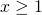We can understand the change of sign when we look at the following easy example. We know that for the numbers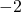en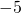the inequality holds: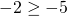However, when we divide (or multiply) both sides by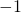we get: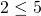The previous procedure could lead to the following inequality: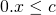Now there are two possibilities.

1.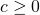In this case all values ofsatisfies the inequality.

2.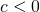In this case no value ofsatisfies the inequality.

We will explain the foregoing by a number of examples.

##### Example 1

Solve: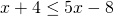We can write this inequality as: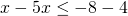so: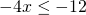We divide both sides by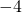. This is a negative number and thus the inequality sign changes: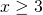##### Eample 2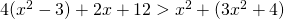At first sight, by the presence of squares ofthis equation does not seem a linear inequality, but yet it is. We remove the parentheses and simplify the result as usual. We can write this inequality as: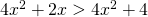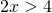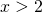We see that we have a linear instead of a quadratic inequality.
We have divided both sides of the inequality by the positive number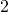and thus the inequality sign does not change.

##### Example 3

Solve: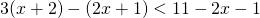We can write this inequality as: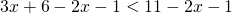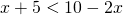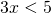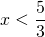We have divided both sides of the inequality by the positive number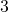and thus the inequality sign does not change.

##### Example 4

Solve: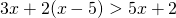We can write this inequality as: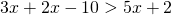or: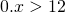No value ofsatisfies this inequality because the left-hand side always equals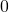which is never greater than.

##### Example 5

Solve: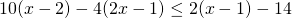We can write this inequality as: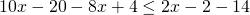or: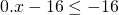or: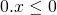All values ofsatisfy this inequality.

0